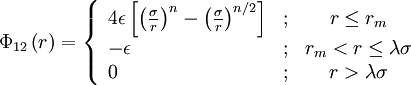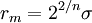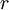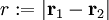# Soft-core square well modelThis article is a 'stub' page, it has no, or next to no, content. It is here at the moment to help form part of the structure of SklogWiki. If you add sufficient material to this article then please remove the {{Stub-general}} template from this page.$\Phi_{12}\left( r \right) = \left\{ \begin{array}{lcc} 4 \epsilon \left[ \left(\frac{\sigma}{r} \right)^{n}- \left( \frac{\sigma}{r}\right)^{n/2} \right] & ; & r \leq r_m \\ - \epsilon & ; &r_m < r \leq \lambda \sigma \\ 0 & ; & r >\lambda \sigma \end{array} \right.$
where$\Phi_{12}(r)$ is the intermolecular pair potential,$r_m = 2^{2/n}\sigma$,$\epsilon$ is the well depth,$r$ is the distance between site 1 and site 2, i.e.$r := |\mathbf{r}_1 - \mathbf{r}_2|$, σ is the hard diameter and λ > 1.